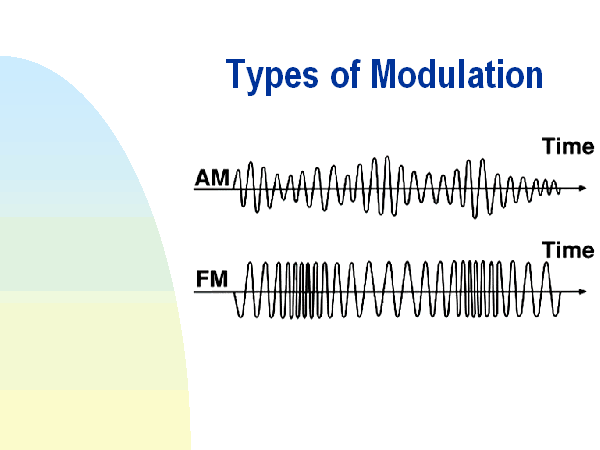# TYPES OF MODULATION

Based on the characteristics of the carrier signal, modulation can be classified as below:

1. Amplitude Modulation.
2. Frequency Modulation.
3. Phase Modulation.THEORY OF AMPLITUDE MODULATION:

• The process of changing the amplitude of the carrier signal in accordance with the modulating signal transmitted, keeping the frequency and phase of the carrier wave unchanged is known as amplitude modulation.

Representation of AM wave:

A typical amplitude modulated signal

• The shape of the AM envelope will be same as that of the information signal or the modulating signal. Frequency representaton of AM wave: (Double side band – full carrier)

Upper side band:

• The band of frequency range between fc and fc+fm is called as upper side band(USB).

Lower side band:

• The band of frequency range between fc and fc-fm is called as lower side band(LSB).

Upper side band frequency:

• Any of the frequency that lies between fc and fc+fm is called as upper side band frequency.

Lower side band frequency:

• Any of the frequency that lies between fc and fc-fm is called as lower side band frequency.

Bandwidth:

• It is the difference between upper side band frequency to the lower side band frequency.
• Upper side band frequency = fc+f
• Lower side band frequency = fc-f

BW= (fc+fm) – (fc-fm)

 BW = 2fm

Therefore,

Coefficent of modulation or modulation index:

• The ratio between the maximum amplitude of the modulating signal to maximum amplitude of the carrier signal is called as modulation index.
 Modulation index, m= Em / Ec

Where,               Em be the maximum amplitude of the modulating signal.

Ec be the maximum amplitude of the modulating signal.

• Value of Em must be less than the value of E
• Hence maximum value of the modulation index will be equal to 1 when Em = E
• Minimum value will be zero.
• If modulation index is higher than 1, then it is called over modulation.Percentage of modulation:

• Whenever the coefficient of modulaton index is expressed in terms of percentage then it is called as percentage of modulation.
 M = (Em / Ec) *100

Modulation signal:

• Consider the upper highest peak value as Vmax=Em-Ec
• Upper lowest peak value as Vmin=Em-Ec
• Downward highest peak value as -Vmax= -Em-Ec

Downward lowest peak value as -Vmin=-Em+Ec

Vmax +Vmin = Em + Ec + Em – Ec

Vmax +Vmin = 2 Em

 Em = (Vmax +Vmin) / 2

Similarly,

Vmax -Vmin = Em + Ec – Em + Ec

Vmax -Vmin = 2 Ec

 Ec = (Vmax -Vmin) / 2

Therefore,

m= Vm / Vc = Em / Ec

Therefore,

 m= (Vmax +Vmin) /(Vmax -Vmin)

 M =((Vmax +Vmin) /(Vmax -Vmin)) *100

Voltage representation of AM wave: (Equation of double side band- full carrier)

From the diagram we can observe the following:

vm(t)=Em Sin wmt

where,  vm(t) be the instantanous value of the modulating signal.

Em be the maximum amplitude of the modulating signal.

wm be the angular frequency of the modulatng signal.

vc(t)=Ec Sin wct

where,  vc(t) be the instantanous value of the carrier signal.

Ec be the maximum amplitude of the carrier signal.

wc be the angular frequency of the carrier signal.

Therefore for amplitude modulated signal,

VAM (t) = (Ec + Em Sin wmt ) Sin wct

VAM (t) = Ec Sin wct + Em Sin wmt Sin wct

VAM (t) = EcSin wct +mEc Sin wmt Sin wct                  [ since m=Em / Ec]

VAM (t) = Ec {Sin wct +m Sin wmt Sin wct}

VAM (t) = Ec {Sin wct +m/2{Cos (wc-wm)t – Cos (wc+wm)t }}

[since SinASinB=1/2(Cos (A-B) – (Cos (A+B))]

VAM (t) = Ec {Sin 2∏fct +m/2{Cos 2∏(fc-fm)t – Cos 2∏(fc+fm)t }}

VAM (t) = Ec Sin 2∏fct – mEc /2{Cos 2∏(fc+fm)t – Cos 2∏(fc-fm)t }}

• Ec be the amplitude of the carrier signal.
• mEc/2 be the amplitude of the USB signal.
• mEc /2 be the amplitude of the LSB signal.
• fc be the frequency of the carrier signal.
• fc+fm be the frequency of the USB signal.
• fc-fm be the frequency of the LSB signal